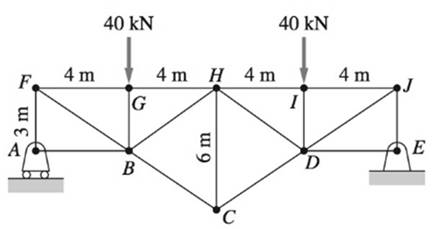Chapter 4, Problem 4.187RP### International Edition---engineerin...

4th Edition
Andrew Pytel And Jaan Kiusalaas
ISBN: 9781305501607
Textbook Problem

# Determine the forces in members GH, BH, and BC of the truss.To determine

Force in members GH, BH and BC.

Explanation

Given information:

To determine the forces on above mechanism:

We use method of sections.

Here we have three unknowns.

Therefore three independent equations need to be used to find unknown forces.

Steps to follow in the equilibrium analysis of a body are:

1. Draw the free body diagram.

2. Write the equilibrium equations.

3. Solve the equations for the unknowns.

Calculation:

FBD of entire section

Assume NA as the reaction force at point A and Ex,Ey as the reaction force components at point E.

The above truss is symmetrical.

Therefore

NA=Ey=40kN

FBD of truss left of section a-a

Assume PGH,PBH,PBC as the forces on member GH, BH and BC respectively.

For the equilibrium of above section, the bending moment about point B is equal to zero.

MB=0

PGH(3m)+NA(4m)=0

Substitute and solve

PGH(3m)+40kN(4m)=0PGH=53.3kN

For the equilibrium of above section, the bending moment about point H is equal to zero.

MH=0

35PBC(8m)+40kN(4m)NA(8m)=0

Substitute and solve

35PBC(

### Still sussing out bartleby?

Check out a sample textbook solution.

See a sample solution

#### The Solution to Your Study Problems

Bartleby provides explanations to thousands of textbook problems written by our experts, many with advanced degrees!

Get Started

## Additional Engineering Solutions

#### What does IADS stand for?

Precision Machining Technology (MindTap Course List)

#### Using Table 16.1, identify the components of the logic system shown in the figure.

Engineering Fundamentals: An Introduction to Engineering (MindTap Course List)

#### What factors are important in a DBMS software selection?

Database Systems: Design, Implementation, & Management

#### What e user applications? Suggest three examples that could boost user productivity.

Systems Analysis and Design (Shelly Cashman Series) (MindTap Course List)

#### Convert P = 5.00 atm into Pa, bar, and psia.

Fundamentals of Chemical Engineering Thermodynamics (MindTap Course List)